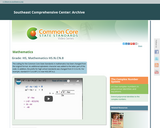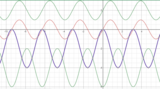Updating search results...

# 5 Results

View
Selected filters:
• Pre-CalculusRating
0.0 stars

Students will understand transformations by first exploring the notion of linearity in an algebraic context. This quickly leads to a return to the study of complex numbers and a study of linear transformations in the complex plane.

Subject:
Math 2
Mathematics
Pre-Calculus
Material Type:
Unit of Study
Provider:
EngageNY
Author:
EngageNY
02/26/2019Rating
0.0 stars

The Common Core State Standards (CCSS) videos are designed to support states, schools, and teachers in the implementation of selected math standards. Each video is an audiovisual resource that focuses on one or more specific standards through examples and illustrations geared to enhancing understanding. The intent of each content-focused video is to clarify the meaning of the individual standard rather than to be a guide on how to teach each standard, although the examples can be adapted for instructional use.

Subject:
Mathematics
Pre-Calculus
Material Type:
Presentation
Provider:
The Southeast Comprehensive Center
Author:
The Southeast Comprehensive Center
02/26/2019Unrestricted Use
CC BY
Rating
0.0 stars

This is an exploration activity for students to visually see the graph of trig functions and understand about sin and cos functions and understand the concept of amplitude.

Subject:
Math 3
Mathematics
Pre-Calculus
Trigonometry
Material Type:
Activity/Lab
Author:
VIJAYALAKSHMI SANKARAN
05/27/2020Unrestricted Use
CC BY
Rating
0.0 stars

This is an exploration activity for students to visually see the graph of trig functions and understand about sin and cos functions and understand the concept of amplitude.Section 2 of this activity allows students to explore the period and vertical shift in trig functions.

Subject:
Math 3
Mathematics
Pre-Calculus
Trigonometry
Material Type:
Activity/Lab
Author:
VIJAYALAKSHMI SANKARAN
05/28/2020Unrestricted Use
CC BY
Rating
0.0 stars

This is an exploration activity for students to visually see the graph of trig functions and understand about sin and cos functions and understand the concept of amplitude.and periods. Thanks to the original author, VIJAYLAKSHMI SANKARAN for sharing and allowing remix access to this resource.

Subject:
Math 3
Mathematics
Pre-Calculus
Trigonometry
Material Type:
Activity/Lab
Self Assessment
Simulation
Author:
Sheree Osbourne-Dixon